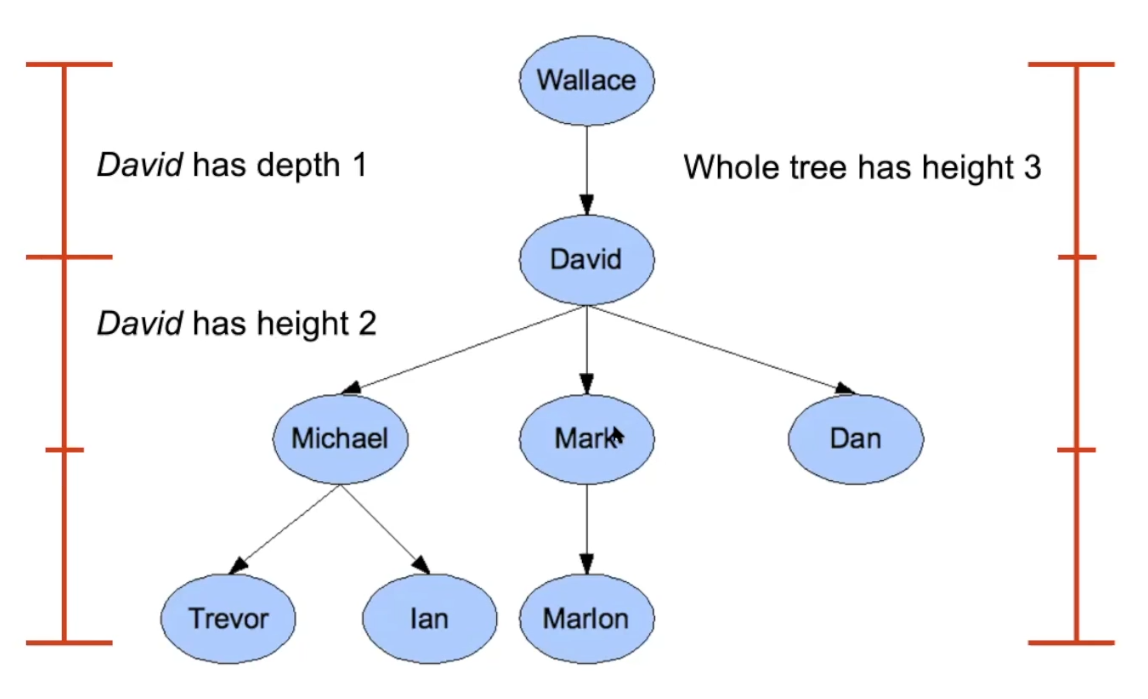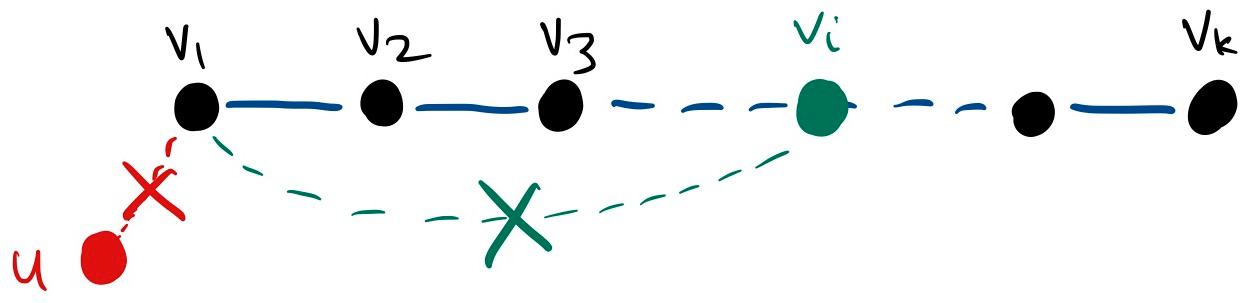Data Structure

# Tree §

A tree is a special kind of directed Graph.

• Root node has no parent
• Each node has 0 or more children

We can sub-categorize trees into:

Leaf nodes are nodes that have no children.

SUPER IMPORTANT PROPERTY: There is only 1 path between any two nodes on the tree. This is not the case with a Graph.

### Depth vs. height §

Height of a node (looking up from leaf node) -> length of the longest path from it down to a leaf

• Leaf node has height 0

Depth of a node (looking down from root) -> length of the path from the root to it

• Root has depth 0### Storing Trees as Arrays §

It is very common to store trees as arrays. The way they are represented is dependent on the tree.

We also saw this in CS240, storing the Heap as an Array.

### From MATH239 §

Definitions

Tree

A tree is a Connected Graph with no cycles.

Forest

A forest is a Graph with no cycles.

Leaf

A leaf in a tree is a vertex of degree 1.

A tree is a “minimally-connected graph”: it is connected, but removing any edge disconnects it.

Theorems and Lemmas There are all these theorems and lemmas that you should be able to prove.

Lemma 5.1.3

Let be vertices in a tree . Then there exists a unique -path in .

Lemma 5.1.4

Every edge in a tree or forest is a bridge.

Theorem 5.1.5.

If is a tree, then .

Theorem 5.1.8

If is a tree with at least two vertices, then it has at least two leaves.Lemma 3

Every tree is bipartite.